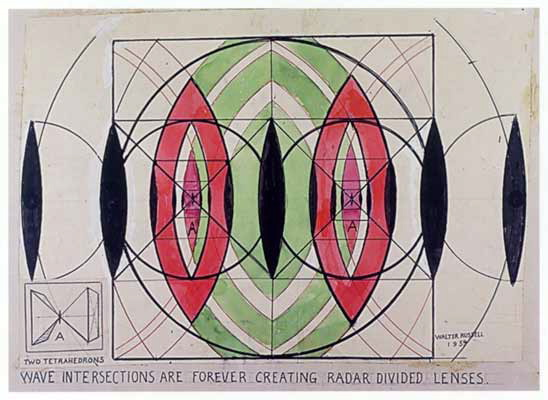bidirectional wave pair

"Electromagnetic waves in vacuum are not force field waves as CEM, classical electromagnetics, prescribes; instead, they are oscillations of potentials and potential gradients. Potentials have a bidirectional electromagnetic wave-pair structure, where the bidirectional wave pairs are phaselocked in a harmonic series. In each wave pair, photons and antiphotons are continually coupling (into spin-2 gravitons) and decoupling. This is where gravitation and electromagnetics are unified. The CEM, classical electromagnetics, notion that singular electromagnetic forces exist in either matter or the vacuum is false; Newton's third law requires that all forces exist in oppositive pairs." [Bearden, The Final Secret of Free Energy]

"There are just gradients of the vacuum potential present in the vacuum. In the vacuum, an electromagnetic wave is actually a wave of the phase locked gradients of the electrostatic scalar potential and of the magnetostatic scalar potential. And each such gradient wave is simultaneously accompanied by its phase conjugate gradient wave, because of Newton's third law." [Bearden, The Final Secret of Free Energy]Figure 3.4 - Focalizing Lenses at nested Cube faces.

Bidirectional Wave Pair
Mate-Pairs
pressure lense
Wave Pair

Bjerknes Effect
Concordance
Figure 3.4 - Focalizing Lenses at nested Cube faces
Gravitational Lens
Harmonic
Law of Attraction
Lens
lense
Mirrors and Lens of Cosmic Cinema
pressure lense
Sympathetic Oscillation
Sympathetic Vibration
The Final Secret of Free Energy